Updating search results...

# 24 Results

View
Selected filters:
• variablesRating
0.0 stars

In this lesson, students write and evaluate algebraic expressions at specific values. They will also use variables to represent numbers and write expressions when solving a real-world or mathematical problem.

Subject:
Mathematics
Material Type:
Activity/Lab
Lesson Plan
Provider:
Utah Education Network
Author:
Utah LessonPlans
02/26/2019Rating
0.0 stars

CK-12 Algebra Explorations is a hands-on series of activities that guides students from Pre-K to Grade 7 through algebraic concepts.

Subject:
Mathematics
Material Type:
Activity/Lab
Lesson Plan
Textbook
Provider:
CK-12 Foundation
Provider Set:
CK-12 FlexBook
Author:
Mary Cavanagh, Carol Findell, Carole Greenes
10/04/2011Rating
0.0 stars

Using tiles to represent variables and constants, learn how to represent and solve algebra problem. Solve equations, substitute in variable expressions, and expand and factor. Flip tiles, remove zero pairs, copy and arrange, and make your way toward a better understanding of algebra.

Subject:
Math 1
Math 2
Math 3
Mathematics
Material Type:
Interactive
Provider:
National Council of Teachers of Mathematics
Author:
Illuminations
02/26/2019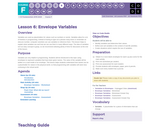Conditional Remix & Share Permitted
CC BY-NC-SA
Rating
0.0 stars

Variables are used as placeholders for values such as numbers or words. Variables allow for a lot of freedom in programming. Instead of having to type out a phrase many times or remember an obscure number, computer scientists can use variables to reference them. This lesson helps to explain what variables are and how we can use them in many different ways. The idea of variables isn't an easy concept to grasp, so we recommend allowing plenty of time for discussion at the end of the lesson.

Subject:
Applied Science
Computer Science
Material Type:
Lesson Plan
Provider:
Code.org
Provider Set:
CS Fundamentals 2019-2020
09/09/2019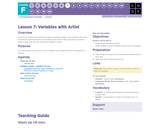Conditional Remix & Share Permitted
CC BY-NC-SA
Rating
0.0 stars

In this lesson, students will explore the creation of repetitive designs using variables in the Artist environment. Students will learn how variables can be used to make code easier to write and easier to read, even when the values don't change at runtime.

Subject:
Applied Science
Computer Science
Material Type:
Lesson Plan
Provider:
Code.org
Provider Set:
CS Fundamentals 2019-2020
09/09/2019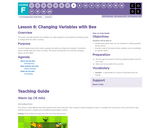Conditional Remix & Share Permitted
CC BY-NC-SA
Rating
0.0 stars

This lesson will help illustrate how variables can make programs more powerful by allowing values to change while the code is running.

Subject:
Applied Science
Computer Science
Material Type:
Lesson Plan
Provider:
Code.org
Provider Set:
CS Fundamentals 2019-2020
09/09/2019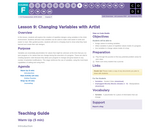Conditional Remix & Share Permitted
CC BY-NC-SA
Rating
0.0 stars

In this lesson, students will explore the creation of repetitive designs using variables in the Artist environment. Students will learn how variables can be used to make code easier to write and easier to read. After guided puzzles, students will end in a freeplay level to show what they have learned and create their own designs.

Subject:
Applied Science
Computer Science
Material Type:
Lesson Plan
Provider:
Code.org
Provider Set:
CS Fundamentals 2019-2020
09/09/2019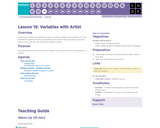Conditional Remix & Share Permitted
CC BY-NC-SA
Rating
0.0 stars

In this lesson, students will explore the creation of repetitive designs using variables in the Artist environment. Students will learn how variables can be used to make code easier to write and easier to read, even when the values don't change at runtime.

Subject:
Applied Science
Computer Science
Material Type:
Lesson Plan
Provider:
Code.org
Provider Set:
CS Fundamentals 2019-2020
09/09/2019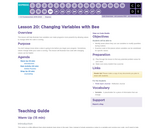Conditional Remix & Share Permitted
CC BY-NC-SA
Rating
0.0 stars

This lesson will help illustrate how variables can make programs more powerful by allowing values to change while the code is running.

Subject:
Applied Science
Computer Science
Material Type:
Lesson Plan
Provider:
Code.org
Provider Set:
CS Fundamentals 2019-2020
09/09/2019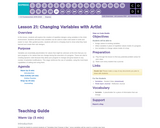Conditional Remix & Share Permitted
CC BY-NC-SA
Rating
0.0 stars

In this lesson, students will explore the creation of repetitive designs using variables in the Artist environment. Students will learn how variables can be used to make code easier to write and easier to read. After guided puzzles, students will end in a freeplay level to show what they have learned and create their own designs.

Subject:
Applied Science
Computer Science
Material Type:
Lesson Plan
Provider:
Code.org
Provider Set:
CS Fundamentals 2019-2020
09/09/2019Rating
0.0 stars

In this lesson, students will estimate capacity and the relationship between several unusally shaped containers. They will verify relationships, express the relationship using variables, and apply the expressions to determine equivalent portions for each standard unit.

Subject:
Mathematics
Material Type:
Activity/Lab
Lesson Plan
Provider:
PBS
Author:
PBS Mathline
02/26/2019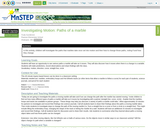Rating
0.0 stars

In this activity students will investigate the paths that marbles take once set into motion and then how to change those paths, noting if and how they change.

Subject:
Physical Science
Science
Material Type:
Activity/Lab
Lesson Plan
Provider:
OER
Author:
Trudy Gunderson, Minnesota Science Teachers Education Project
02/26/2019Conditional Remix & Share Permitted
CC BY-NC
Rating
0.0 stars
Rating
0.0 stars
Subject:
Mathematics
Material Type:
Full Course
Provider:
Pearson
11/02/2020Conditional Remix & Share Permitted
CC BY-NC
Rating
0.0 stars

Expressions

Type of Unit: Concept

Prior Knowledge

Students should be able to:

Write and evaluate simple expressions that record calculations with numbers.
Use parentheses, brackets, or braces in numerical expressions and evaluate expressions with these symbols.
Interpret numerical expressions without evaluating them.

Lesson Flow

Students learn to write and evaluate numerical expressions involving the four basic arithmetic operations and whole-number exponents. In specific contexts, they create and interpret numerical expressions and evaluate them. Then students move on to algebraic expressions, in which letters stand for numbers. In specific contexts, students simplify algebraic expressions and evaluate them for given values of the variables. Students learn about and use the vocabulary of algebraic expressions. Then they identify equivalent expressions and apply properties of operations, such as the distributive property, to generate equivalent expressions. Finally, students use geometric models to explore greatest common factors and least common multiples.

Subject:
Algebra
Mathematics
Provider:
PearsonConditional Remix & Share Permitted
CC BY-NC
Rating
0.0 stars

Students play an Expressions Game in which they describe expressions to their partners using the vocabulary of expressions: term, coefficient, exponent, constant, and variable. Their partners try to write the correct expressions based on the descriptions.Key ConceptsMathematical expressions have parts, and these parts have names. These names allow us to communicate with others in a precise way.A variable is a symbol (usually a letter) in an expression that can be replaced by a number.A term is a number, a variable, or a product of numbers and variables. Terms are separated by the operator symbols + (plus) and – (minus).A coefficient is a symbol (usually a number) that multiplies the variable in an algebraic expression.An exponent tells how many copies of a number or variable are multiplied together.A constant is a number. In an expression, it can be a constant term or a constant coefficient. In the expression 2x + 3, 2 is a constant coefficient and 3 is a constant term.Goals and Learning ObjectivesIdentify parts of an expression using appropriate mathematical vocabulary.Write expressions that fit specific descriptions (for example, the expression is the sum of two terms each with a different variable).

Subject:
Algebra
Material Type:
Lesson Plan
Author:
Pearson
11/02/2020Conditional Remix & Share Permitted
CC BY-NC
Rating
0.0 stars

Four full-year digital course, built from the ground up and fully-aligned to the Common Core State Standards, for 7th grade Mathematics. Created using research-based approaches to teaching and learning, the Open Access Common Core Course for Mathematics&nbsp;is designed with student-centered learning in mind, including activities for students to develop valuable 21st century skills and academic mindset.

Subject:
Mathematics
Material Type:
Full Course
Provider:
Pearson
11/02/2020Conditional Remix & Share Permitted
CC BY-NC
Rating
0.0 stars

Algebraic Reasoning

Type of Unit: Concept

Prior Knowledge

Students should be able to:

Add, subtract, multiply, and divide rational numbers.
Evaluate expressions for a value of a variable.
Use the distributive property to generate equivalent expressions including combining like terms.
Understand solving an equation or inequality as a process of answering a question: which values from a specified set, if any, make the equation or inequality true?
Write and solve equations of the form x+p=q and px=q for cases in which p, q, and x are non-negative rational numbers.
Understand and graph solutions to inequalities x&lt;c or x&gt;c.
Use equations, tables, and graphs to represent the relationship between two variables.
Relate fractions, decimals, and percents.
Solve percent problems included those involving percent of increase or percent of decrease.

Lesson Flow

This unit covers all of the Common Core State Standards for Expressions and Equations in Grade 7. Students extend what they learned in Grade 6 about evaluating expressions and using properties to write equivalent expressions. They write, evaluate, and simplify expressions that now contain both positive and negative rational numbers. They write algebraic expressions for problem situations and discuss how different equivalent expressions can be used to represent different ways of solving the same problem. They make connections between various forms of rational numbers. Students apply what they learned in Grade 6 about solving equations such as x+2=6 or 3x=12 to solving equations such as 3x+6=12 and 3(x−2)=12. Students solve these equations using formal algebraic methods. The numbers in these equations can now be rational numbers. They use estimation and mental math to estimate solutions. They learn how solving linear inequalities differs from solving linear equations and then they solve and graph linear inequalities such as −3x+4&lt;12. Students use inequalities to solve real-world problems, solving the problem first by arithmetic and then by writing and solving an inequality. They see that the solution of the algebraic inequality may differ from the solution to the problem.

Subject:
Algebra
Mathematics
Provider:
Pearson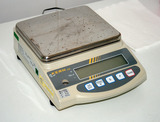Conditional Remix & Share Permitted
CC BY-NC
Rating
0.0 stars

Students use algebraic expressions and equations to represent rules of thumb involving measurement. They use properties of operations and the relationships between fractions, decimals, and percents to write equivalent expressions.Key ConceptsExpressions and equations are different. An expression is a number, a variable, or a combination of numbers and variables. Some examples of expressions are:74x5a + b3(2m + 1)In Grade 7, the focus is on linear expressions. A linear expression is a sum of terms that are either rational numbers or a rational number times a variable (with an exponent of either 0 or 1). If an expression contains a variable, it is called an algebraic expression. To evaluate an expression, each variable is replaced with a given value.Equivalent expressions are expressions for which a given value can be substituted for each variable and the value of the expressions are the same.An equation is a statement that two expressions are equal. An equation can be true or false. To solve an equation, students find the value of the variable that makes the equation true.Students solve an equation that involves finding 10% of a number. They see that finding 10% of the number is the same as finding 0.1 of the number, or finding 110 of the number.Goals and Learning ObjectivesWrite expressions and equations to represent real-world situations.Evaluate expressions for given values of a variable.Use properties of operations to write equivalent expressions.Solve one-step equations.Check the solution to an equation.

Subject:
Algebra
Material Type:
Lesson Plan
Author:
Pearson
11/02/2020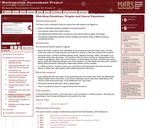Only Sharing Permitted
CC BY-NC-ND
Rating
0.0 stars

This lesson unit is intended to help you assess how well students use algebra in context, and in particular, how well students: explore relationships between variables in everyday situations; find unknown values from known values; find relationships between pairs of unknowns, and express these as tables and graphs; and find general relationships between several variables, and express these in different ways by rearranging formulae.

Subject:
Algebra
Mathematics
Material Type:
Assessment
Lesson Plan
Provider:
Shell Center for Mathematical Education
Provider Set:
Mathematics Assessment Project (MAP)
07/31/2019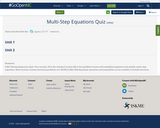Conditional Remix & Share Permitted
CC BY-NC
Rating
0.0 stars

8.EE.7 Solving Equations Quiz. Two versions. M is the standard version M1 is the modified version with simplified negatives and smaller multi-step equations. Both versions contain fractional problems too.

NCM1.A-REI.3 Solving linear equations and inequalities in one variable to include fractions.

Subject:
Algebra
Geometry
Mathematics
Material Type:
Assessment